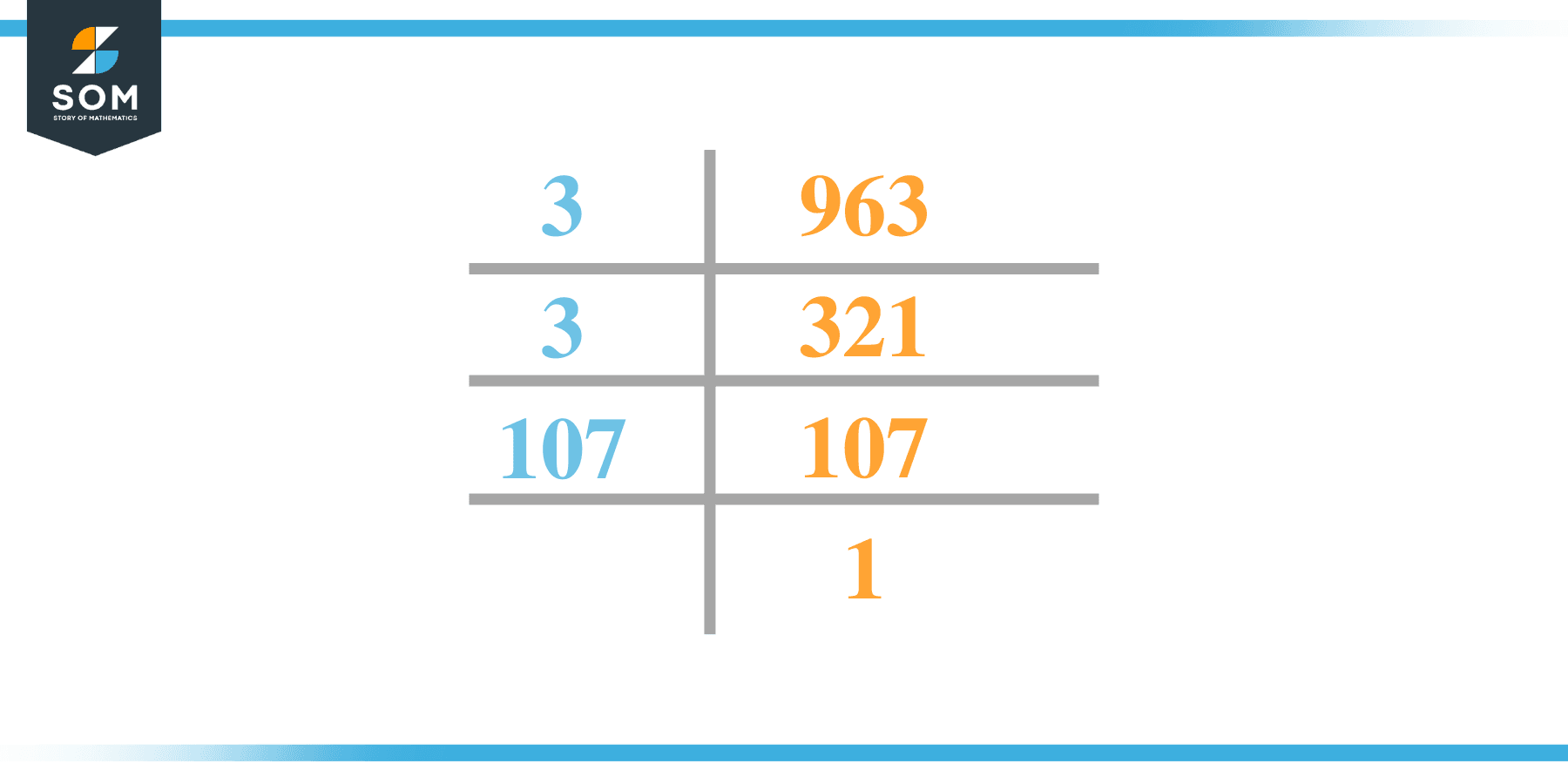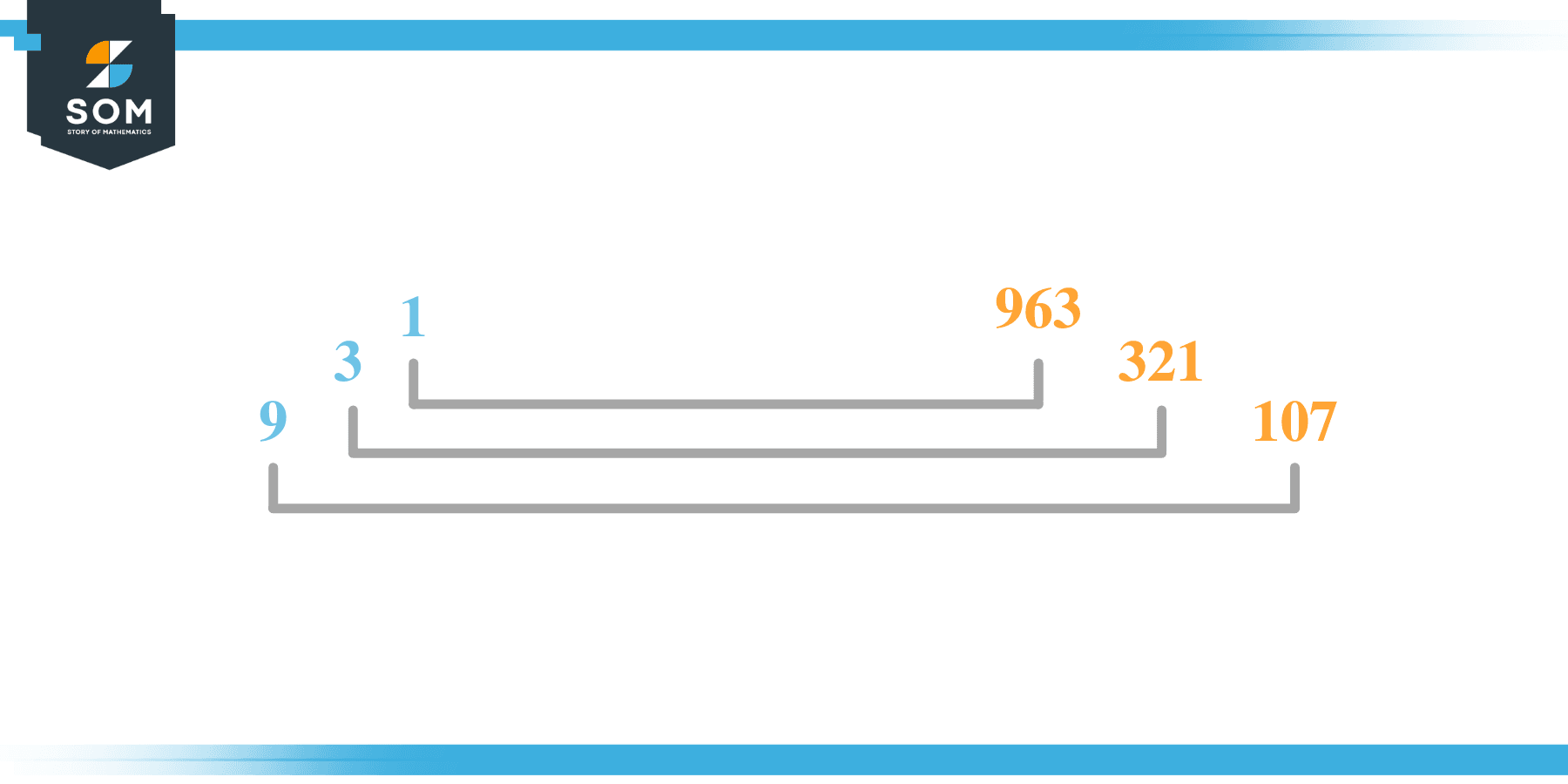# Factors of 963: Prime Factorization, Methods, and Examples

The number 963 has 6 positive factors and 6 negative factors. These factors are the additive inverse of each other which means that the sum of positive and negative factors of 936 is equal to zero.All these 12 numbers give a remainder equal to zero on division with 963.

### Factors of 963

Here are the factors of number 963.

Factors of 963: 1, 3, 9, 107, 321, and 963

### Negative Factors of 963

The negative factors of 963 are similar to its positive aspects, just with a negative sign.

Negative Factors of 963: -1, -3, -9, -107, -321, and -963

### Prime Factorization of 963

The prime factorization of 963 is the way of expressing its prime factors in the product form.

Prime Factorization: 3$^2$ x 107

In this article, we will learn about the factors of 963 and how to find them using various techniques such as upside-down division, prime factorization, and factor tree.

## What Are the Factors of 936?

The factors of 963 are 1, 3, 9, 107, 321, and 963. These numbers are the factors as they do not leave any remainder when divided by 963.

The factors of 963 are classified as prime numbers and composite numbers. The prime factors of the number 963 can be determined using the prime factorization technique.

## How To Find the Factors of 963?

You can find the factors of 963 by using the rules of divisibility. The divisibility rule states that any number, when divided by any other natural number, is said to be divisible by the number if the quotient is the whole number and the resulting remainder is zero.

To find the factors of 963, create a list containing the numbers that are exactly divisible by 963 with zero remainders. One important thing to note is that 1 and 963 are the 963’s factors as every natural number has 1 and the number itself as its factor.

1 is also called the universal factor of every number. The factors of 963 are determined as follows:

$\dfrac{963}{1} = 963$

$\dfrac{963}{3} = 321$

$\dfrac{963}{9} = 107$

$\dfrac{963}{107} = 9$

$\dfrac{963}{321} = 3$

$\dfrac{963}{963} = 1$

Therefore, 1, 3, 9, 107, 321, and 963 are the factors of 963.

### Total Number of Factors of 963

For 963, there are 6 positive factors and 6 negative ones. So in total, there are 12 factors of 963.

To find the total number of factors of the given number, follow the procedure mentioned below:

1. Find the factorization/prime factorization of the given number.
2. Demonstrate the prime factorization of the number in the form of exponent form.
3. Add 1 to each of the exponents of the prime factor.
4. Now, multiply the resulting exponents together. This obtained product is equivalent to the total number of factors of the given number.

By following this procedure, the total number of factors of X is given as:

Factorization of 963 is 1 x 3$^2$ x 107.

The exponent of 1 and 107 is 1 whereas the exponent of 3 is 2.

Adding 1 to each and multiplying them together results in 12.

Therefore, the total number of factors of 963 is 12. 6 is positive, and 6 factors are negative.

### Important Notes

Here are some essential points that must be considered while finding the factors of any given number:

• The factor of any given number must be a whole number.
• The factors of the number cannot be in the form of decimals or fractions.
• Factors can be positive as well as negative.
• Negative factors are the additive inverse of the positive factors of a given number.
• The factor of a number cannot be greater than that number.
• Every even number has 2 as its prime factor, the smallest prime factor.

## Factors of 963 by Prime FactorizationThe number 963 is a composite number. Prime factorization is a valuable technique for finding the number’s prime factors and expressing the number as the product of its prime factors.

Before finding the factors of 963 using prime factorization, let us find out what prime factors are. Prime factors are the factors of any given number that are only divisible by 1 and themselves.

To start the prime factorization of 963, start dividing by its most minor prime factor. First, determine that the given number is either even or odd. If it is an even number, then 2 will be the smallest prime factor.

Continue splitting the quotient obtained until 1 is received as the quotient. The prime factorization of 963 can be expressed as:

963 = 3$^2$ x 107

## Factors of 963 in PairsThe factor pairs are the duplet of numbers that, when multiplied together, result in the factorized number. Factor pairs can be more than one depending on the total number of factors given.

For 963, the factor pairs can be found as:

1 x 963 = 963

3 x 321 = 963

9 x 107 = 963

The possible factor pairs of 963 are given as (1, 963), (3, 321), and (9, 107).

All these numbers in pairs, when multiplied, give 963 as the product.

The negative factor pairs of 963 are given as:

-1 x -963 = 963

-3 x -321 = 963

-9 x -107 = 963

It is important to note that in negative factor pairs, the minus sign has been multiplied by the minus sign, due to which the resulting product is the original positive number. Therefore, -1, -3, -9, -107, -321, and -963 are called negative factors of 963.

The list of all the factors of 963, including positive as well as negative numbers, is given below.

Factor list of 963: 1, -1, 3, -3, 9, -9, 107, -107, 321, -321, 963, and -963

## Factors of 963 Solved Examples

To better understand the concept of factors, let’s solve some examples.

### Example 1

How many factors of 963 are there?

### Solution

The total number of Factors of 963 is 6.

Factors of 963 are 1, 3, 9, 107, 321, and 963.

### Example 2

Find the factors of 963 using prime factorization.

### Solution

The prime factorization of 963 is given as:

963 $\div$ 3 = 321

321 $\div$ 3 = 107

107 $\div$ 107 = 1

So the prime factorization of 963 can be written as:

3$^2$ x 107 = 963